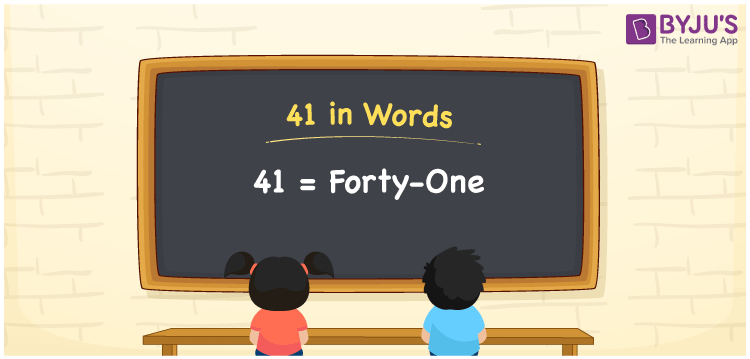# 41 in Words

41 in words is written as Forty-one. In both the International System of Numerals and the Indian System of Numerals, 41 is written as Forty-one. The number 41 is a Cardinal Number as it could represent some quantity. For example, “there are 41 students present in the class”. Clearly, 41 quantifies the number of students.

 41 in words Forty-one Forty-one in Number 41

## 41 in English Words

41 in English words is read as “Forty-one”.## How to Write 41 in Words?

To write 41 in words, we shall use the place value chart. In the place value chart, put 4 in the tens place and 1 in the ones place. Let us make a place value chart to write the number 41 in words.

 Tens Ones 4 1

Thus, we can write the expanded form as

4 × Ten + 1 × One

= 4 × 10 + 1 × 1

= 40 + 1

= 41

= Forty-one.

41 is a natural number, the successor of 40 and the predecessor of 42.

41 in words – Forty-one

• Is 41 an odd number? – Yes
• Is 41 an even number? – No
• Is 41 a perfect square number? – No
• Is 41 a perfect cube number? – No
• Is 41 a prime number? – Yes
• Is 41 a composite number? – No

## Frequently Asked Questions on 41 in Words

Q1

### How to write 41 in words?

41 in words is written as Forty-one.
Q2

### How to write 41 in the International and Indian System of Numerals?

In both, the system of numerals, 41 in words, is written as Forty-one.
Q3

### How to write 41 in a place value chart?

In the place value chart, write 4 in the tens place and 1 in the ones place.